Dr. J's Maths.com
Where the techniques of Maths
are explained in simple terms.

Calculus - Integration - Differentiate ... hence find (basic function) problems.
Test Yourself 1.

 1. (i) Differentiate (3x2 + x)3 (ii) Hence find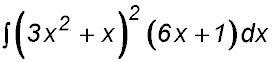. 2. (i) Differentiate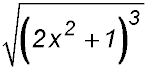. (ii) Hence evaluate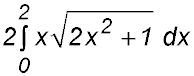. Answer.(ii) Integral = 26/3. 3. (i) Differentiate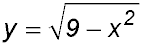with respect to x. (ii) Hence or otherwise find4. (i) Differentiate (x2 + 1)3. (ii) Hence find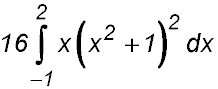. Answer.(ii) Integral = 312. 5. (i) Differentiate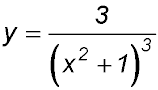. (ii) Hence find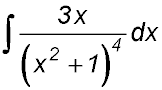. 6.